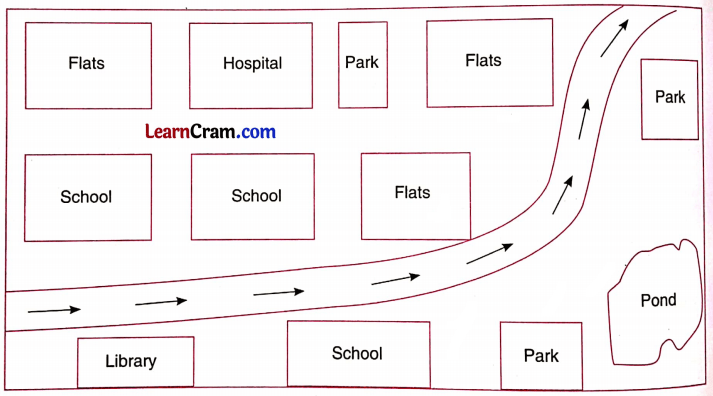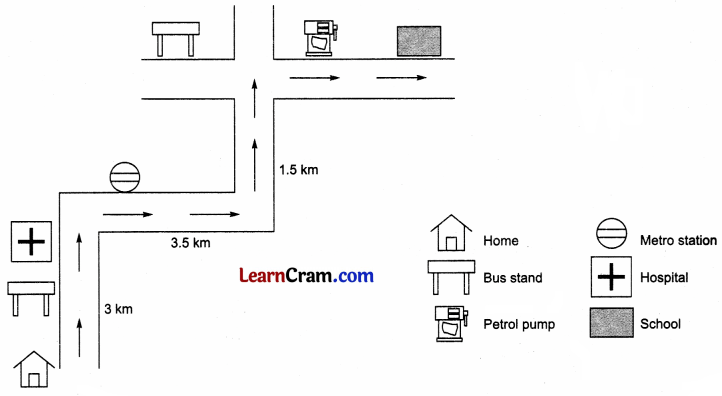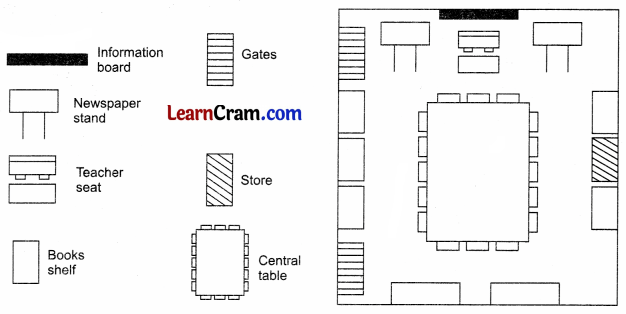# DAV Class 8 Maths Chapter 14 Worksheet 9 Solutions

The DAV Maths Book Class 8 Solutions Pdf and DAV Class 8 Maths Chapter 14 Worksheet 9 Solutions of Mensuration offer comprehensive answers to textbook questions.

## DAV Class 8 Maths Ch 14 WS 9 Solutions

Question 1.
Look at the map of a city given below:Colour the map as directed:
Blue – Water
Pink – Flats
Green – Parks
Yellow – Library
Orange – School
Purple – Hospital
Solution:Question 2.
Draw a map of the route from your house to your school showing important landmarks.
Solution:
Maps may vary from student to student. The sample map is shown below.Question 3.
Draw a map of your classroom using the proper scale and symbols for different objects.
Solution:
Maps may vary. A sample map is shown below.
Scale: 1 cm = 2.5 m
Symbols for landmarks.Question 4.
Draw a map of your school library in detail.
Solution:
Maps may vary. A sample map is shown below.### DAV Class 8 Maths Chapter 14 Value Based Questions

Question 1.
Raman a painter, always donated one-fifth of his income to an orphanage. Once he got a contract to paint a house having three rooms of dimensions 10 m × 12 m × 3.5 m, 8 m × 6 m × 3.5 m, and 7 m × 5 m × 3.5 m. He charged ₹ 30 per m2 for his work.
(i) How much money did Raman earn?
(ii) Find the amount of money he gave to the orphanage.
(iii) What feature of Raman’s personality would you appreciate and why?
Solution:
(i) Area of rooms to be painted = Area of four walls + Area of ceiling
= 2(l + b) × h + l × b
= {2(12 + 10) × 3.5 + 12 × 10} + {2(8 + 6) × 3.5 + 8 × 6} + {2(7 + 5) × 3.5 + 7 × 5}
= (2 × 22 × 3.5 + 120) + (2 × 14 × 3.5 + 48) + (2 × 12 × 3.5 + 35)
= (154 + 120) + (98 + 48) + (84 + 35)
= 274 + 146 + 119
= 539 m2
Money earned by Raman = ₹ 30 × 539 = ₹ 16170

(ii) The amount given by Raman to orphanage = one-fifth of his income
= $$\frac{1}{5}$$ × ₹ 16170
= ₹ 3234Question 2.
For ‘Beti Bachao, Beti Padhao’, a rally was organized by a school. Monal wrote the slogan on a trapezium-shaped cardboard having the length of parallel sides 60 cm and 90 cm respectively and the distance between them was 50 cm.
(i) Find the area of the trapezium.Area of ABCD = $$\frac{1}{2}$$ × height × sum of parallel sides
= $$\frac{1}{2}$$ × 50 × (60 + 90)
= $$\frac{1}{2}$$ × 50 × 150